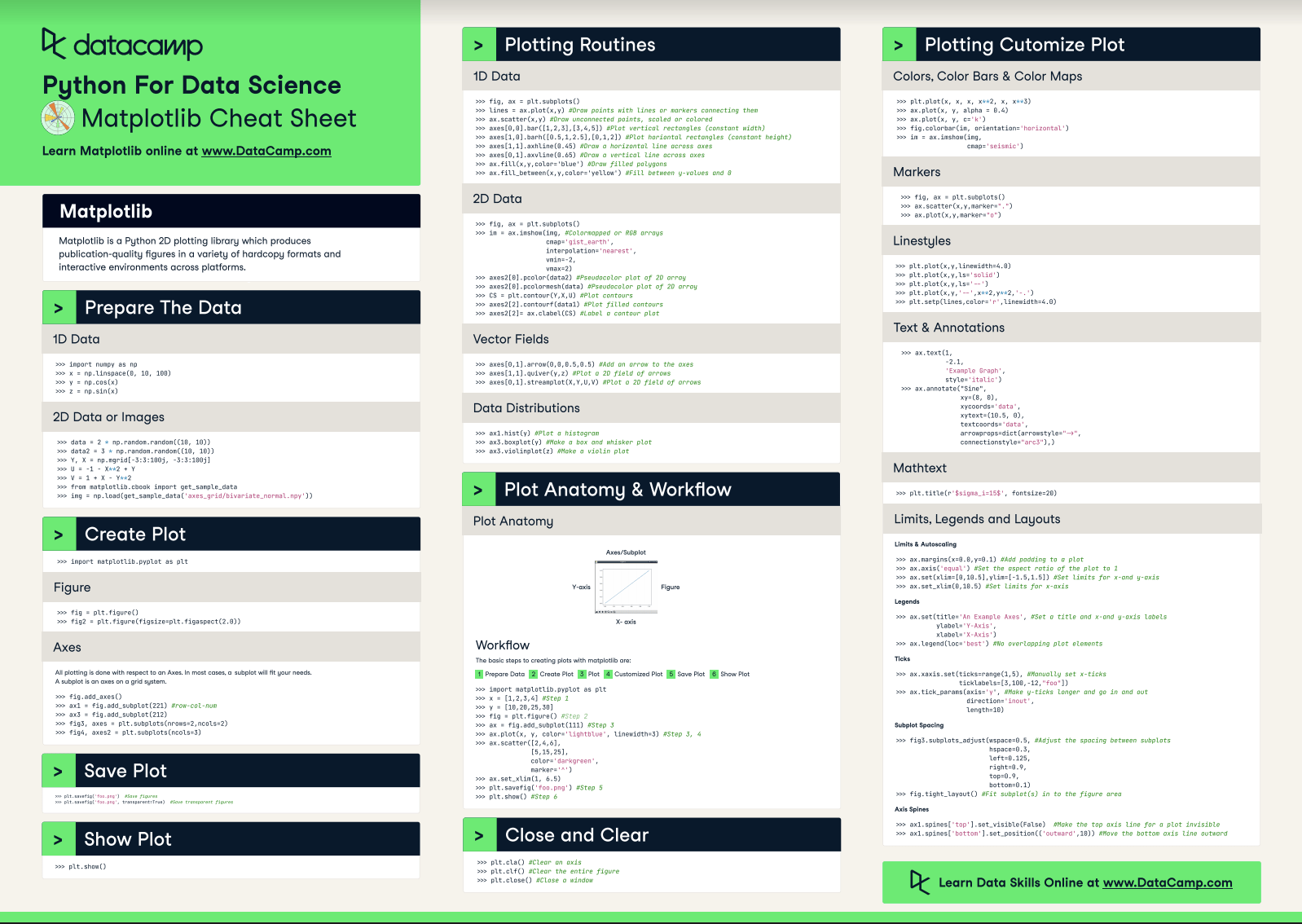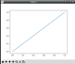HomeCheat sheetsPython

# Matplotlib Cheat Sheet: Plotting in Python

This Matplotlib cheat sheet introduces you to the basics that you need to plot your data with Python and includes code samples.
Jun 2021  · 6 min read

Data visualization and storytelling with your data are essential skills that every data scientist needs to communicate insights gained from analyses effectively to any audience out there.

For most beginners, the first package that they use to get in touch with data visualization and storytelling is, naturally, Matplotlib: it is a Python 2D plotting library that enables users to make publication-quality figures. But, what might be even more convincing is the fact that other packages, such as Pandas, intend to build more plotting integration with Matplotlib as time goes on.

However, what might slow down beginners is the fact that this package is pretty extensive. There is so much that you can do with it and it might be hard to still keep a structure when you're learning how to work with Matplotlib.

DataCamp has created a Matplotlib cheat sheet for those who might already know how to use the package to their advantage to make beautiful plots in Python, but that still want to keep a one-page reference handy. Of course, for those who don't know how to work with Matplotlib, this might be the extra push be convinced and to finally get started with data visualization in Python.

(By the way, if you want to get started with this Python package, you might want to consider our Matplotlib tutorial.)

You'll see that this cheat sheet presents you with the six basic steps that you can go through to make beautiful plots.

Check out the infographic by clicking on the button below:Have this cheat sheet at your fingertips

With this handy reference, you'll familiarize yourself in no time with the basics of Matplotlib: you'll learn how you can prepare your data, create a new plot, use some basic plotting routines to your advantage, add customizations to your plots, and save, show and close the plots that you make.

What might have looked difficult before will definitely be more clear once you start using this cheat sheet! Use it in combination with the Matplotlib Gallery, the documentation and our tutorial.

Also, don't miss out on our other cheat sheets for data science that cover SciPyNumpyScikit-LearnBokehPandas and the Python basics.

Matplotlib

Matplotlib is a Python 2D plotting library which produces publication-quality figures in a variety of hardcopy formats and interactive environments across platforms.

## Prepare the Data

### 1D Data

``````>>> import numpy as np
>>> x = np.linspace(0, 10, 100)
>>> y = np.cos(x)
>>> z = np.sin(x)``````

### 2D Data or Images

``````>>> data = 2 * np.random.random((10, 10))
>>> data2 = 3 * np.random.random((10, 10))
>>> Y, X = np.mgrid[-3:3:100j, -3:3:100j]
>>> U = 1 X** 2 + Y
>>> V = 1 + X Y**2
>>> from matplotlib.cbook import get_sample_data

## Create Plot

``>>> import matplotlib.pyplot as plt``

### Figure

``````>>> fig = plt.figure()
>>> fig2 = plt.figure(figsize=plt.figaspect(2.0))``````

### Axes

``````>>> fig.add_axes()
>>> fig3, axes = plt.subplots(nrows=2,ncols=2)
>>> fig4, axes2 = plt.subplots(ncols=3)``````

## Save Plot

``````>>> plt.savefig('foo.png') #Save figures
>>> plt.savefig('foo.png',  transparent=True) #Save transparent figures``````

## Show Plot

``>>> plt.show()``

## 1D Data

``````>>> fig, ax = plt.subplots()
>>> lines = ax.plot(x,y) #Draw points with lines or markers connecting them
>>> ax.scatter(x,y) #Draw unconnected points, scaled or colored
>>> axes[0,0].bar([1,2,3],[3,4,5]) #Plot vertical rectangles (constant width)
>>> axes[1,0].barh([0.5,1,2.5],[0,1,2]) #Plot horiontal rectangles (constant height)
>>> axes[1,1].axhline(0.45) #Draw a horizontal line across axes
>>> axes[0,1].axvline(0.65) #Draw a vertical line across axes
>>> ax.fill(x,y,color='blue') #Draw filled polygons
>>> ax.fill_between(x,y,color='yellow') #Fill between y values and 0``````

### 2D Data

``````>>> fig, ax = plt.subplots()
>>> im = ax.imshow(img, #Colormapped or RGB arrays
cmap= 'gist_earth',
interpolation= 'nearest',
vmin=-2,
vmax=2)
>>> axes2.pcolor(data2) #Pseudocolor plot of 2D array
>>> axes2.pcolormesh(data) #Pseudocolor plot of 2D array
>>> CS = plt.contour(Y,X,U) #Plot contours
>>> axes2.contourf(data1) #Plot filled contours
>>> axes2= ax.clabel(CS) #Label a contour plot``````

### Vector Fields

``````>>> axes[0,1].arrow(0,0,0.5,0.5) #Add an arrow to the axes
>>> axes[1,1].quiver(y,z) #Plot a 2D field of arrows
>>> axes[0,1].streamplot(X,Y,U,V) #Plot a 2D field of arrows``````

### Data Distributions

``````>>> ax1.hist(y) #Plot a histogram
>>> ax3.boxplot(y) #Make a box and whisker plot
>>> ax3.violinplot(z)  #Make a violin plot``````

## Plot Anatomy & Workflow

### Plot Anatomy

y-axisx-axis

### Workflow

The basic steps to creating plots with matplotlib are:

1 Prepare Data
2 Create Plot
3 Plot
4 Customized Plot
5 Save Plot
6 Show Plot

``````>>> import matplotlib.pyplot as plt
>>> x = [1,2,3,4]  #Step 1
>>> y = [10,20,25,30]
>>> fig = plt.figure() #Step 2
>>> ax = fig.add_subplot(111) #Step 3
>>> ax.plot(x, y, color= 'lightblue', linewidth=3)  #Step 3, 4
>>> ax.scatter([2,4,6],
[5,15,25],
color= 'darkgreen',
marker= '^' )
>>> ax.set_xlim(1, 6.5)
>>> plt.savefig('foo.png' ) #Step 5
>>> plt.show() #Step 6``````

## Close and Clear

``````>>> plt.cla()  #Clear an axis
>>> plt.clf(). #Clear the entire figure
>>> plt.close(). #Close a window``````

## Plotting Customize Plot

### Colors, Color Bars & Color Maps

``````>>> plt.plot(x, x, x, x**2, x, x** 3)
>>> ax.plot(x, y, alpha = 0.4)
>>> ax.plot(x, y, c= 'k')
>>> fig.colorbar(im, orientation= 'horizontal')
>>> im = ax.imshow(img,
cmap= 'seismic' )``````

### Markers

``````>>> fig, ax = plt.subplots()
>>> ax.scatter(x,y,marker= ".")
>>> ax.plot(x,y,marker= "o")``````

### Linestyles

``````>>> plt.plot(x,y,linewidth=4.0)
>>> plt.plot(x,y,ls= 'solid')
>>> plt.plot(x,y,ls= '--')
>>> plt.plot(x,y,'--' ,x**2,y**2,'-.' )
>>> plt.setp(lines,color= 'r',linewidth=4.0)``````

### Text & Annotations

``````>>> ax.text(1,
-2.1,
'Example Graph',
style= 'italic' )
>>> ax.annotate("Sine",
xy=(8, 0),
xycoords= 'data',
xytext=(10.5, 0),
textcoords= 'data',
arrowprops=dict(arrowstyle= "->",
connectionstyle="arc3"),)``````

### Mathtext

``>>> plt.title(r '\$sigma_i=15\$', fontsize=20)``

### Limits, Legends and Layouts

Limits & Autoscaling

``````>>> ax.margins(x=0.0,y=0.1) #Add padding to a plot
>>> ax.axis('equal')  #Set the aspect ratio of the plot to 1
>>> ax.set(xlim=[0,10.5],ylim=[-1.5,1.5])  #Set limits for x-and y-axis
>>> ax.set_xlim(0,10.5) #Set limits for x-axis``````

Legends

``````>>> ax.set(title= 'An Example Axes',  #Set a title and x-and y-axis labels
ylabel= 'Y-Axis',
xlabel= 'X-Axis')
>>> ax.legend(loc= 'best')  #No overlapping plot elements``````

Ticks

``````>>> ax.xaxis.set(ticks=range(1,5),  #Manually set x-ticks
ticklabels=[3,100, 12,"foo" ])
>>> ax.tick_params(axis= 'y', #Make y-ticks longer and go in and out
direction= 'inout',
length=10)``````

Subplot Spacing

``````>>> fig3.subplots_adjust(wspace=0.5,   #Adjust the spacing between subplots
hspace=0.3,
left=0.125,
right=0.9,
top=0.9,
bottom=0.1)
>>> fig.tight_layout() #Fit subplot(s) in to the figure area``````

Axis Spines

``````>>> ax1.spines[ 'top'].set_visible(False) #Make the top axis line for a plot invisible
>>> ax1.spines['bottom' ].set_position(( 'outward',10))  #Move the bottom axis line outward``````
Related

### Building Your Data Science Portfolio with DataCamp Workspace (Part 1)

Learn how to build a comprehensive data science portfolio by exploring examples different examples, mastering tips to make your work stand out, and utilizing the DataCamp Workspace effectively to showcase your results.9 min

### Pandas 2.0: What’s New and Top Tips

Dive into pandas 2.0, the latest update of the essential data analysis library, with new features like PyArrow integration, nullable data types, and non-nanosecond datetime resolution for better performance and efficiency.### Building a Safer Internet with Data Science

Learn the key drivers of a data strategy that helps ensure online safety and consumer protection with Richard Davis, the Chief Data Officer at Ofcom, the UK’s government-approved regulatory and competition authority.

### Conda Cheat Sheet

In this cheat sheet, learn all about the basics of working with Conda. From managing and installing packages, to working with channels & environments, learn the fundamentals of the conda package management tool suite.

### Matplotlib time series line plot

This tutorial explores how to create and customize time series line plots in matplotlib.

### Visualizing Data in Excel